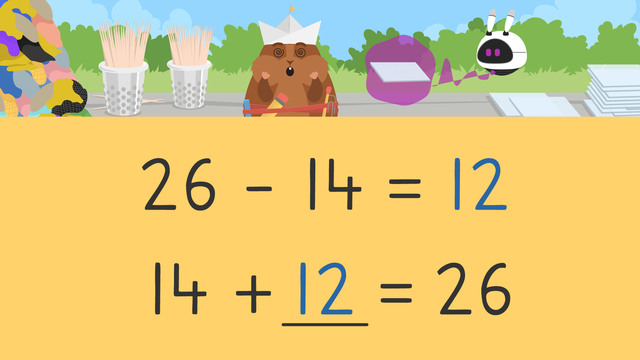Rating

Ø 5.0 / 2 ratings

The authorsTeam Digital
CCSS.MATH.CONTENT.2.OA.A.1

## Basics on the topicSubtract Using Addition

### Content

When solving subtraction problems, you can us addition to subtract. There are many tools and strategies to solve addition problems, one of which is using a number line! When using addition to solve subtraction problems...

• Start by creating a new addition equation using what you know from the subtraction equation.

• Next, put the smaller addend on the left side of the number line.

• Then, count up the bigger number in the equation. You can do this by counting forward small amounts, like by fives or tens!

• Finally, find your sum by looking at how much you counted to get to the second number. Remember, the answer to the addition equation is also the answer to your subtraction equation!